# How to Calculate and Solve for Average Repeat Unit Molecular Weight for Co-Polymers | Polymer

The average repeat unit molecular weight for co-polymers is represented by the image below.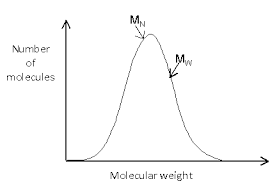To compute for average repeat unit molecular weight for co-polymers, one essential parameter is needed and the parameter is Numbers of co-polymers (n). By determining the number of co-polymers, four parameters is needed to get your answer so the parameters are Mole Fraction (f1), Molecular Weight (M1), Mole Fraction (f2) and Molecular Weight (M2).

The formula for calculating the average repeat unit molecular weight for co-polymers:

m’ = ΣfiMi

Let’s solve an example;
Find the average repeat unit molecular weight for co-polymers when the mole fraction(s) is 8,2 and the molecular weight(s) is 4,6.

This implies that;

m’ = ΣfiMi

Where:

m’ = Average Repeat Unit Molecular Weight for Co-Polymers
fi = Mole Fraction(s)
Mi = Molecular Weight(s)

m’ = (8)(4) + (2)(6)
m’ = (32) + (12)
m’ = 44

Therefore, the average repeat unit molecular weight for co-polymers is 44.

Nickzom Calculator – The Calculator Encyclopedia is capable of calculating the average repeat unit molecular weight for co-polymers.

To get the answer and workings of the average repeat unit molecular weight for co-polymers using the Nickzom Calculator – The Calculator Encyclopedia. First, you need to obtain the app.

You can get this app via any of these means:

You can also try the demo version via https://www.nickzom.org/calculator

Apple (Paid) – https://itunes.apple.com/us/app/nickzom-calculator/id1331162702?mt=8
Once, you have obtained the calculator encyclopedia app, proceed to the Calculator Map, then click on Materials and Metallurgical under Engineering.Now, Click on Polymer under Materials and MetallurgicalNow, Click on Average Repeat Unit Molecular Weight for Co-polymers under Polymer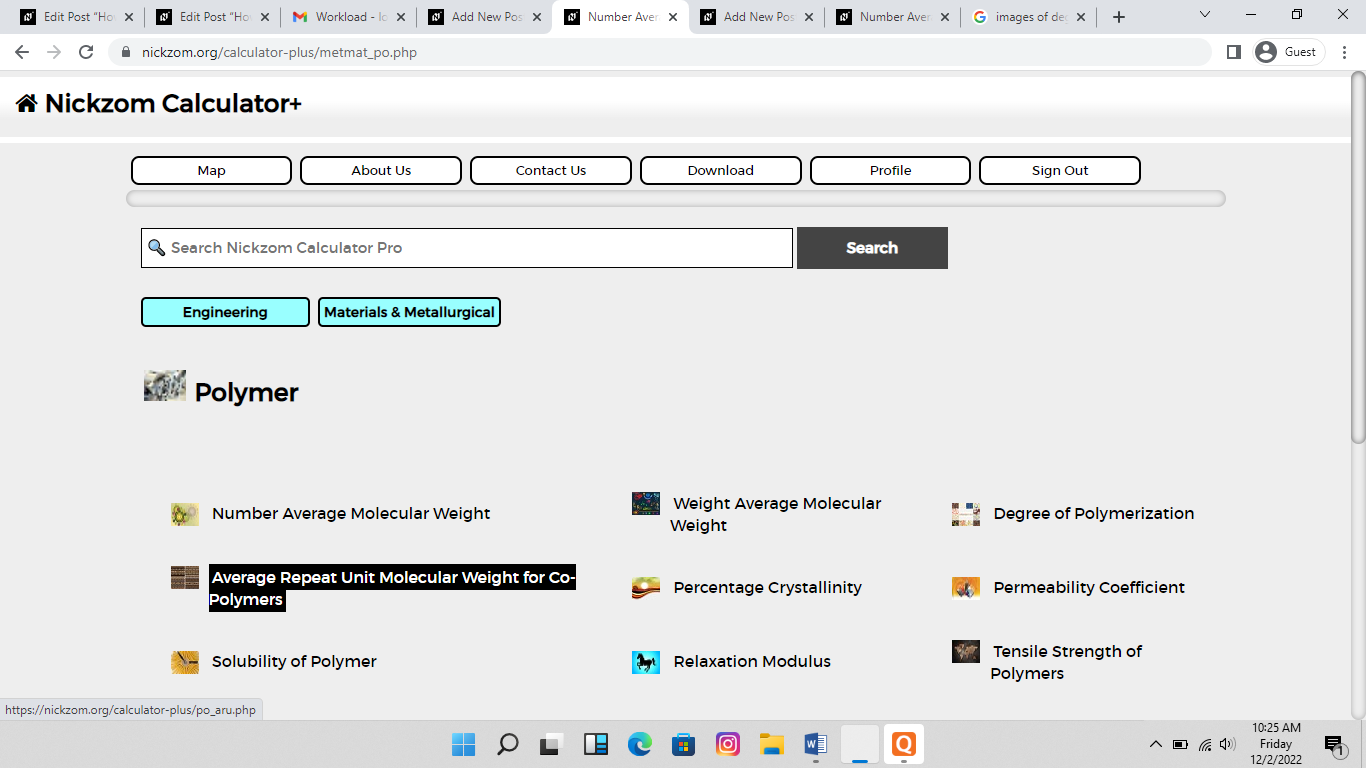The screenshot below displays the page or activity to enter your values, to get the answer for the average repeat unit molecular weight for co-polymers according to the respective parameter which is the Mole Fraction(s) (f1,f2) and Molecular Weight(s) (M1,M2).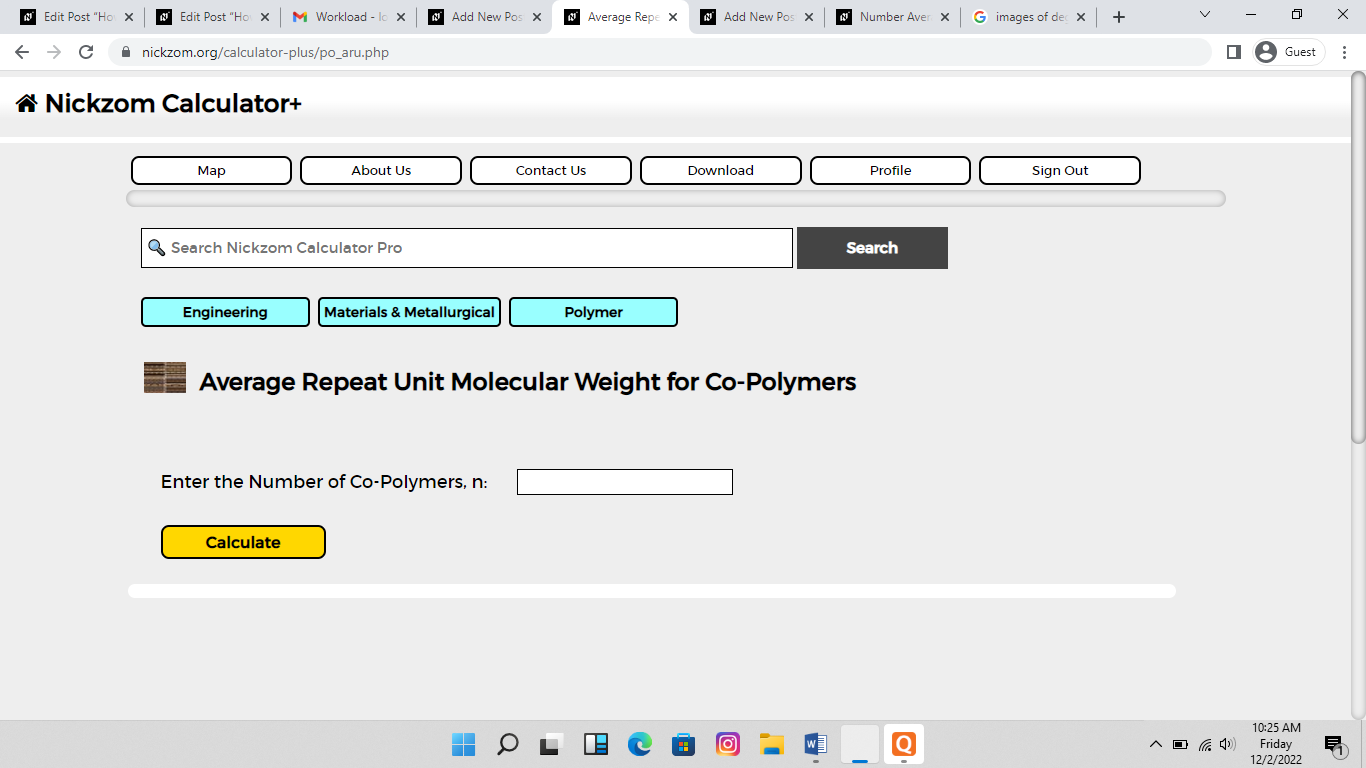Now, enter the values appropriately and accordingly for the parameters as required by the Mole Fraction(s) (f1,f2) is 8,2 and Molecular Weight(s) (M1,M2) is 4,6.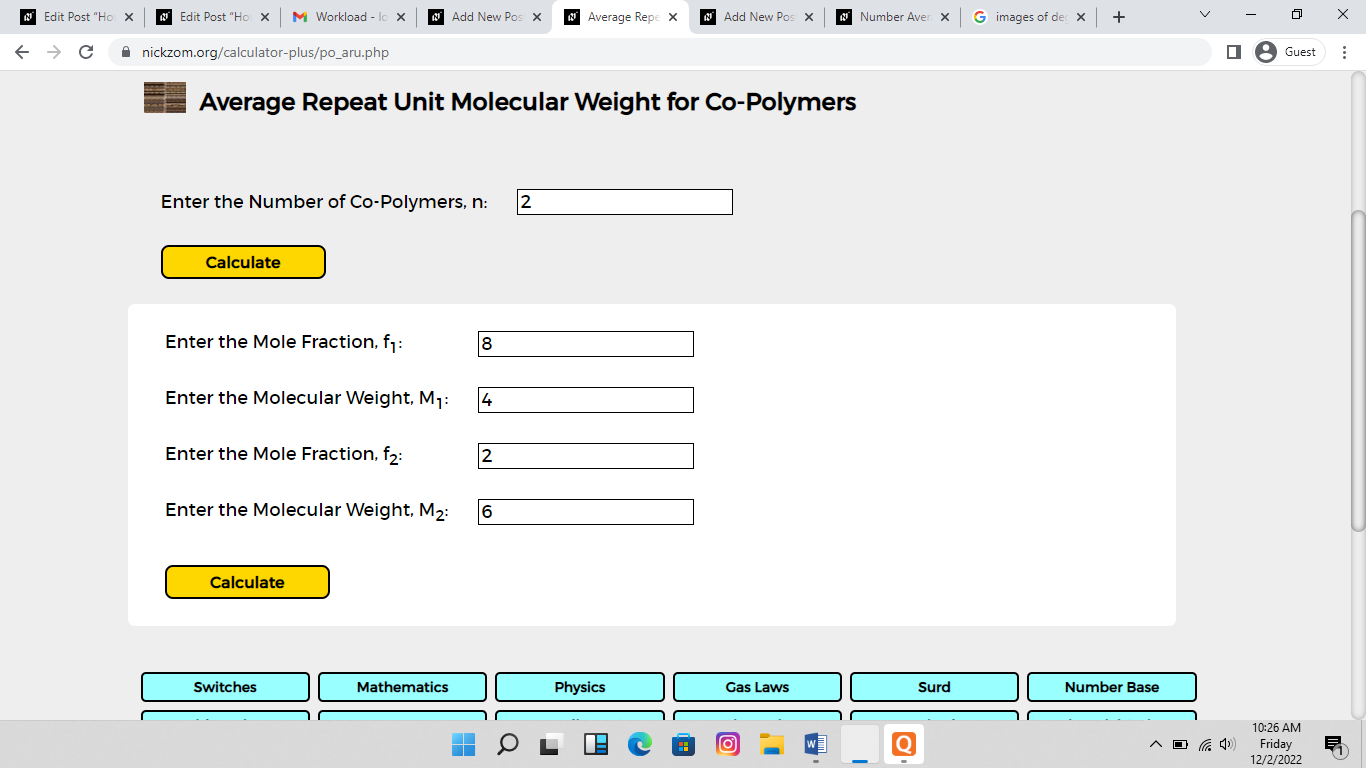Finally, Click on Calculate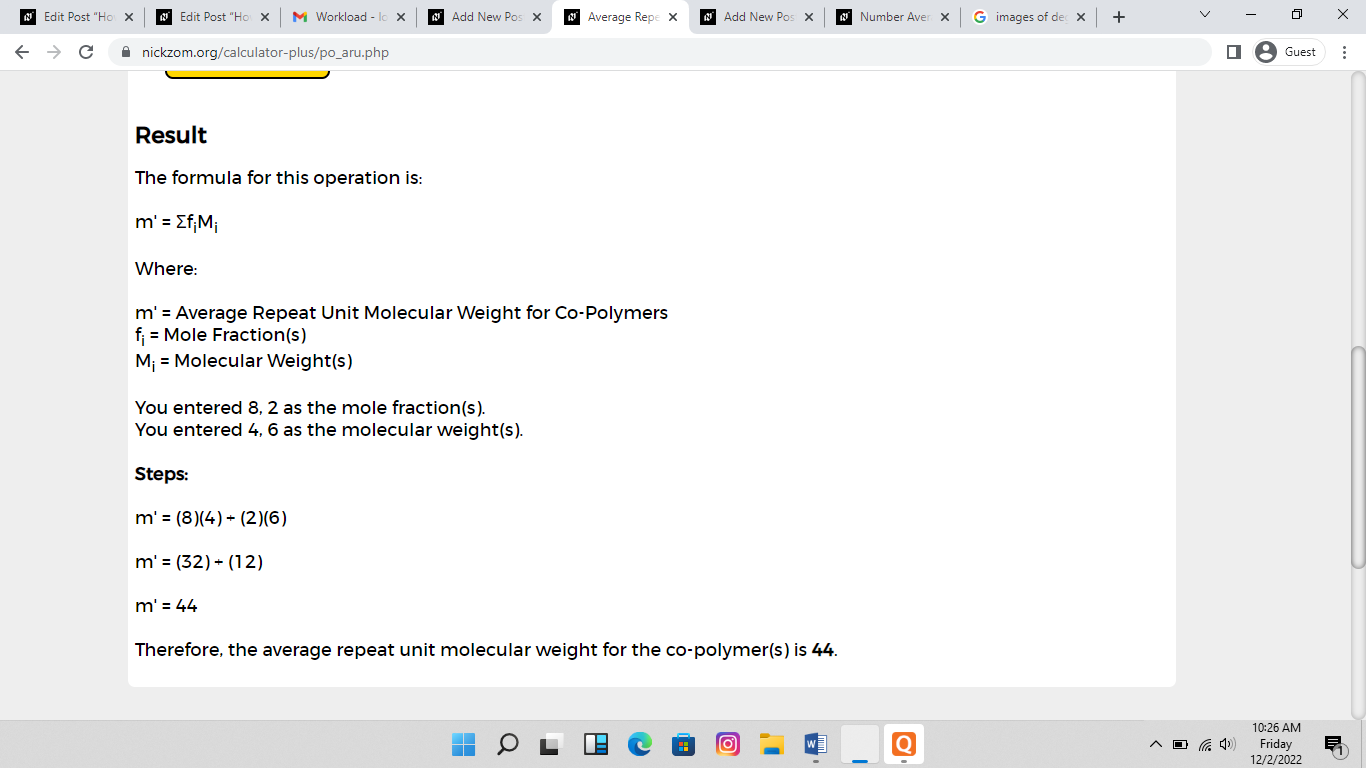As you can see from the screenshot above, Nickzom Calculator– The Calculator Encyclopedia solves for the average repeat unit molecular weight for co-polymers and presents the formula, workings and steps too.